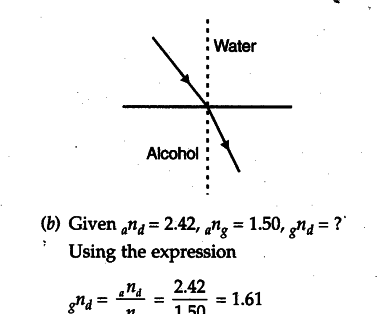# Water has refractive index 1.33 and alcohol has refractive index 1.36

(a) Water has refractive index 1.33 and alcohol has refractive index 1.36. Which of the two medium is optically denser? Give reason for your answer. Draw a ray diagram to show the path of a ray of light passing obliquely from water to alcohol.
(b) The absolute refractive index of diamond is 2.42 and the absolute refractive index of glass is 1.50. Find the refractive index of diamond with respect to glass.

(a) Alcohol is optically denser than water. This is because greater the refractive index more dense the liquid/ material.
The diagram is as shown :